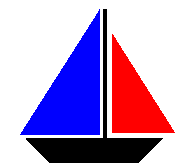Cleave Books
Puzzle Trio 10

 28 A man is aged 71 and his daughter is 34. (a) What age will the daughter be when she is half the age of her father? (b) What age was the man when he was three times the age of his daughter?

29. Here is a magic square using the numbers 1 to 16 (with no repeats) arranged so the magic total of the numbers along every row, column and diagonal is 34.
It is possible, by replacing ONLY ONE of the numbers and then re-arranging all of them, to make the magic total 36.
To do that - which number must be replaced by what?
 1 14 15 4 8 11 10 5 12 7 6 9 13 2 3 16

 30Both the sails of my boat are in the shape of a right-angled triangle. They are of different areas, each being an exact number of square metres. All their edge lengths are different as well, but each is an exact number of metres. However, they do have one property in common. The area of each is numerically equal to its perimeter. What is the area of each sail?